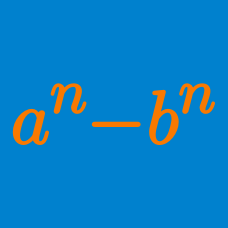Algebra

# Algebraic Identities

The polynomial $9-4x^2-20xy-25y^2$ can be factorized as $$(3+ax+by)(3+cx+dy),$$ where $$a,$$ $$b,$$ $$c$$ and $$d$$ are real numbers. What is the value of $$abcd$$?

What is the value of $$a+b+c$$ if \begin{align} & x^2+8y^2-6xy-2yz+zx+4x-16y+4z \\ &= (x-ay+b)(x-cy+z)? \end{align}

If $$a$$, $$b$$ and $$c$$ are real numbers and $(x^2+2x)^2-79(x^2+2x)-80=(x+a)^2(x+b)(x+c)$ is an identity in $$x$$, what is the value of $$a+b+c$$?

What is the value of $$a+b+c$$ if \begin{align} & (x^2+3x)^2+10x^2+30x-56 \\ &= (x-1)(x+a)(x^2+bx+c)? \end{align}

What is the value of $$a+b+c$$ if \begin{align} & x^2+5xy+4y^2+5x+23y-6 \\ &= (x+ay-b)(x+y+c)? \end{align}

×